# User’s guide¶

Solph is an oemof-package, designed to create and solve linear or mixed-integer linear optimization problems. The package is based on pyomo. To create an energy system model generic and specific components are available. To get started with solph, checkout the examples in the Examples section.

This User’s guide provides a user-friendly introduction into oemof-solph, which includes small examples and nice illustrations. However, the functionality of oemof-solph go beyond the content of this User’s guide section. So, if you want to know all details of a certain component or a function, please go the API Reference. There, you will find a detailed and complete description of all oemof-solph modules.

## How can I use solph?¶

To use solph you have to install oemof and at least one solver (see Installation), which can be used together with pyomo (e.g. CBC, GLPK, Gurobi, Cplex). See the pyomo installation guide for all supported solver. You can test it by executing one of the existing examples (see Examples, or directly oemof’s example repository). Be aware that the examples require the CBC solver but you can change the solver name in the example files to your solver.

Once the example work you are close to your first energy model.

### Handling of Warnings¶

The solph library is designed to be as generic as possible to make it possible to use it in different use cases. This concept makes it difficult to raise Error or Warnings because sometimes untypical combinations of parameters are allowed even though they might be wrong in over 99% of the use cases.

Therefore, a SuspiciousUsageWarning was introduced. This warning will warn you if you do something untypical. If you are sure that you know what you are doing you can switch the warning off.

### Set up an energy system¶

In most cases an EnergySystem object is defined when we start to build up an energy system model. The EnergySystem object will be the main container for the model.

To define an EnergySystem we need a Datetime index to define the time range and increment of our model. An easy way to this is to use the pandas time_range function. The following code example defines the year 2011 in hourly steps. See pandas date_range guide for more information.

import pandas as pd
my_index = pd.date_range('1/1/2011', periods=8760, freq='H')


This index can be used to define the EnergySystem:

import oemof.solph as solph
my_energysystem = solph.EnergySystem(timeindex=my_index)


Now you can start to add the components of the network.

### Add components to the energy system¶

After defining an instance of the EnergySystem class you have to add all nodes you define in the following to your EnergySystem.

Basically, there are two types of nodes - components and buses. Every Component has to be connected with one or more buses. The connection between a component and a bus is the flow.

All solph components can be used to set up an energy system model but you should read the documentation of each component to learn about usage and restrictions. For example it is not possible to combine every component with every flow. Furthermore, you can add your own components in your application (see below) but we would be pleased to integrate them into solph if they are of general interest (see Feature requests and feedback).

An example of a simple energy system shows the usage of the nodes for real world representations:The figure shows a simple energy system using the four basic network classes and the Bus class. If you remove the transmission line (transport 1 and transport 2) you get two systems but they are still one energy system in terms of solph and will be optimised at once.

There are different ways to add components to an energy system. The following line adds a bus object to the energy system defined above.

my_energysystem.add(solph.Bus())


It is also possible to assign the bus to a variable and add it afterwards. In that case it is easy to add as many objects as you like.

my_bus1 = solph.Bus()
my_bus2 = solph.Bus()


Therefore it is also possible to add lists or dictionaries with components but you have to dissolve them.

# add a list



#### Bus¶

All flows into and out of a bus are balanced. Therefore an instance of the Bus class represents a grid or network without losses. To define an instance of a Bus only a unique label is necessary. If you do not set a label a random label is used but this makes it difficult to get the results later on.

To make it easier to connect the bus to a component you can optionally assign a variable for later use.

solph.Bus(label='natural_gas')
electricity_bus = solph.Bus(label='electricity')


Note

See the Bus class for all parameters and the mathematical background.

#### Flow¶

The flow class has to be used to connect. An instance of the Flow class is normally used in combination with the definition of a component. A Flow can be limited by upper and lower bounds (constant or time-dependent) or by summarised limits. For all parameters see the API documentation of the Flow class or the examples of the nodes below. A basic flow can be defined without any parameter.

solph.Flow()


Oemof has different types of flows but you should be aware that you cannot connect every flow type with every component.

Note

See the Flow class for all parameters and the mathematical background.

#### Components¶

Components are divided in three categories. Basic components (solph.network), additional components (solph.components) and custom components (solph.custom). The custom section was created to lower the entry barrier for new components. Be aware that these components are in an experimental state. Let us know if you have used and tested these components. This is the first step to move them to the components section.

See Solph components for a list of all components.

The typical optimisation of an energy system in solph is the dispatch optimisation, which means that the use of the sources is optimised to satisfy the demand at least costs. Therefore, variable cost can be defined for all components. The cost for gas should be defined in the gas source while the variable costs of the gas power plant are caused by operating material. You can deviate from this scheme but you should keep it consistent to make it understandable for others.

Costs do not have to be monetary costs but could be emissions or other variable units.

Furthermore, it is possible to optimise the capacity of different components (see Using the investment mode).

# set up a simple least cost optimisation
om = solph.Model(my_energysystem)

# solve the energy model using the CBC solver
om.solve(solver='cbc', solve_kwargs={'tee': True})


If you want to analyse the lp-file to see all equations and bounds you can write the file to you disc. In that case you should reduce the timesteps to 3. This will increase the readability of the file.

# set up a simple least cost optimisation
om = solph.Model(my_energysystem)

# write the lp file for debugging or other reasons
om.write('path/my_model.lp', io_options={'symbolic_solver_labels': True})


If you want to analyse your results, you should first dump your EnergySystem instance, otherwise you have to run the simulation again.

my_energysystem.results = processing.results(om)
my_energysystem.dump('my_path', 'my_dump.oemof')


If you need the meta results of the solver you can do the following:

my_energysystem.results['main'] = processing.results(om)
my_energysystem.results['meta'] = processing.meta_results(om)
my_energysystem.dump('my_path', 'my_dump.oemof')


To restore the dump you can simply create an EnergySystem instance and restore your dump into it.

import oemof.solph as solph
my_energysystem = solph.EnergySystem()
my_energysystem.restore('my_path', 'my_dump.oemof')
results = my_energysystem.results

# If you use meta results do the following instead of the previous line.
results = my_energysystem.results['main']
meta = my_energysystem.results['meta']


If you call dump/restore without any parameters, the dump will be stored as ‘es_dump.oemof’ into the ‘.oemof/dumps/’ folder created in your HOME directory.

See Handling Results to learn how to process, plot and analyse the results.

## Solph components¶

### Sink (basic)¶

A sink is normally used to define the demand within an energy model but it can also be used to detect excesses.

The example shows the electricity demand of the electricity_bus defined above. The ‘my_demand_series’ should be sequence of normalised valueswhile the ‘nominal_value’ is the maximum demand the normalised sequence is multiplied with. Giving ‘my_demand_series’ as parameter ‘fix’ means that the demand cannot be changed by the solver.

solph.Sink(label='electricity_demand', inputs={electricity_bus: solph.Flow(
fix=my_demand_series, nominal_value=nominal_demand)})


In contrast to the demand sink the excess sink has normally less restrictions but is open to take the whole excess.

solph.Sink(label='electricity_excess', inputs={electricity_bus: solph.Flow()})


Note

The Sink class is only a plug and provides no additional constraints or variables.

### Source (basic)¶

A source can represent a pv-system, a wind power plant, an import of natural gas or a slack variable to avoid creating an in-feasible model.

While a wind power plant will have an hourly feed-in depending on the weather conditions the natural_gas import might be restricted by maximum value (nominal_value) and an annual limit (summed_max). As we do have to pay for imported gas we should set variable costs. Comparable to the demand series an fix is used to define a fixed the normalised output of a wind power plant. Alternatively, you might use max to allow for easy curtailment. The nominal_value sets the installed capacity.

solph.Source(
label='import_natural_gas',
outputs={my_energysystem.groups['natural_gas']: solph.Flow(
nominal_value=1000, summed_max=1000000, variable_costs=50)})

solph.Source(label='wind', outputs={electricity_bus: solph.Flow(
fix=wind_power_feedin_series, nominal_value=1000000)})


Note

The Source class is only a plug and provides no additional constraints or variables.

### Transformer (basic)¶

An instance of the Transformer class can represent a node with multiple input and output flows such as a power plant, a transport line or any kind of a transforming process as electrolysis, a cooling device or a heat pump. The efficiency has to be constant within one time step to get a linear transformation. You can define a different efficiency for every time step (e.g. the thermal powerplant efficiency according to the ambient temperature) but this series has to be predefined and cannot be changed within the optimisation.

A condensing power plant can be defined by a transformer with one input (fuel) and one output (electricity).

b_gas = solph.Bus(label='natural_gas')
b_el = solph.Bus(label='electricity')

solph.Transformer(
label="pp_gas",
inputs={bgas: solph.Flow()},
outputs={b_el: solph.Flow(nominal_value=10e10)},
conversion_factors={electricity_bus: 0.58})


A CHP power plant would be defined in the same manner but with two outputs:

b_gas = solph.Bus(label='natural_gas')
b_el = solph.Bus(label='electricity')
b_th = solph.Bus(label='heat')

solph.Transformer(
label='pp_chp',
inputs={b_gas: Flow()},
outputs={b_el: Flow(nominal_value=30),
b_th: Flow(nominal_value=40)},
conversion_factors={b_el: 0.3, b_th: 0.4})


A CHP power plant with 70% coal and 30% natural gas can be defined with two inputs and two outputs:

b_gas = solph.Bus(label='natural_gas')
b_coal = solph.Bus(label='hard_coal')
b_el = solph.Bus(label='electricity')
b_th = solph.Bus(label='heat')

solph.Transformer(
label='pp_chp',
inputs={b_gas: Flow(), b_coal: Flow()},
outputs={b_el: Flow(nominal_value=30),
b_th: Flow(nominal_value=40)},
conversion_factors={b_el: 0.3, b_th: 0.4,
b_coal: 0.7, b_gas: 0.3})


A heat pump would be defined in the same manner. New buses are defined to make the code cleaner:

b_el = solph.Bus(label='electricity')
b_th_low = solph.Bus(label='low_temp_heat')
b_th_high = solph.Bus(label='high_temp_heat')

# The cop (coefficient of performance) of the heat pump can be defined as
# a scalar or a sequence.
cop = 3

solph.Transformer(
label='heat_pump',
inputs={b_el: Flow(), b_th_low: Flow()},
outputs={b_th_high: Flow()},
conversion_factors={b_el: 1/cop,
b_th_low: (cop-1)/cop})


If the low-temperature reservoir is nearly infinite (ambient air heat pump) the low temperature bus is not needed and, therefore, a Transformer with one input is sufficient.

Note

See the Transformer class for all parameters and the mathematical background.

### ExtractionTurbineCHP (component)¶

The ExtractionTurbineCHP inherits from the Transformer (basic) class. Like the name indicates, the application example for the component is a flexible combined heat and power (chp) plant. Of course, an instance of this class can represent also another component with one input and two output flows and a flexible ratio between these flows, with the following constraints: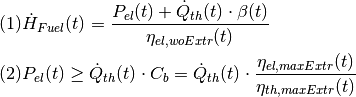where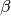is defined as: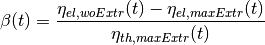where the first equation is the result of the relation between the input flow and the two output flows, the second equation stems from how the two output flows relate to each other, and the symbols used are defined as follows (with Variables (V) and Parameters (P)):

symbol attribute type explanation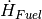flow[i, n, t] V fuel input flow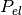flow[n, main_output, t] V electric power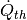flow[n, tapped_output, t] V thermal outputmain_flow_loss_index[n, t] P power loss index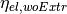conversion_factor_full_condensation[n, t] P
electric efficiency
without heat extraction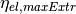conversion_factors[main_output][n, t] P
electric efficiency
with max heat extraction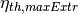conversion_factors[tapped_output][n, t] P
thermal efficiency with
maximal heat extraction

These constraints are applied in addition to those of a standard Transformer. The constraints limit the range of the possible operation points, like the following picture shows. For a certain flow of fuel, there is a line of operation points, whose slope is defined by the power loss factor(in some contexts also referred to as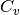). The second constraint limits the decrease of electrical power and incorporates the backpressure coefficient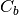.For now, ExtractionTurbineCHP instances must have one input and two output flows. The class allows the definition of a different efficiency for every time step that can be passed as a series of parameters that are fixed before the optimisation. In contrast to the Transformer, a main flow and a tapped flow is defined. For the main flow you can define a separate conversion factor that applies when the second flow is zero (conversion_factor_full_condensation).

solph.ExtractionTurbineCHP(
label='variable_chp_gas',
inputs={b_gas: solph.Flow(nominal_value=10e10)},
outputs={b_el: solph.Flow(), b_th: solph.Flow()},
conversion_factors={b_el: 0.3, b_th: 0.5},
conversion_factor_full_condensation={b_el: 0.5})


The key of the parameter ‘conversion_factor_full_condensation’ defines which of the two flows is the main flow. In the example above, the flow to the Bus ‘b_el’ is the main flow and the flow to the Bus ‘b_th’ is the tapped flow. The following plot shows how the variable chp (right) schedules it’s electrical and thermal power production in contrast to a fixed chp (left). The plot is the output of an example in the example repository.Note

See the ExtractionTurbineCHP class for all parameters and the mathematical background.

### GenericCHP (component)¶

With the GenericCHP class it is possible to model different types of CHP plants (combined cycle extraction turbines, back pressure turbines and motoric CHP), which use different ranges of operation, as shown in the figure below.Combined cycle extraction turbines: The minimal and maximal electric power without district heating (red dots in the figure) define maximum load and minimum load of the plant. Beta defines electrical power loss through heat extraction. The minimal thermal condenser load to cooling water and the share of flue gas losses at maximal heat extraction determine the right boundary of the operation range.

solph.components.GenericCHP(
label='combined_cycle_extraction_turbine',
fuel_input={bgas: solph.Flow(
H_L_FG_share_max=[0.19 for p in range(0, periods)])},
electrical_output={bel: solph.Flow(
P_max_woDH=[200 for p in range(0, periods)],
P_min_woDH=[80 for p in range(0, periods)],
Eta_el_max_woDH=[0.53 for p in range(0, periods)],
Eta_el_min_woDH=[0.43 for p in range(0, periods)])},
heat_output={bth: solph.Flow(
Q_CW_min=[30 for p in range(0, periods)])},
Beta=[0.19 for p in range(0, periods)],
back_pressure=False)


For modeling a back pressure CHP, the attribute back_pressure has to be set to True. The ratio of power and heat production in a back pressure plant is fixed, therefore the operation range is just a line (see figure). Again, the P_min_woDH and P_max_woDH, the efficiencies at these points and the share of flue gas losses at maximal heat extraction have to be specified. In this case “without district heating” is not to be taken literally since an operation without heat production is not possible. It is advised to set Beta to zero, so the minimal and maximal electric power without district heating are the same as in the operation point (see figure). The minimal thermal condenser load to cooling water has to be zero, because there is no condenser besides the district heating unit.

solph.components.GenericCHP(
label='back_pressure_turbine',
fuel_input={bgas: solph.Flow(
H_L_FG_share_max=[0.19 for p in range(0, periods)])},
electrical_output={bel: solph.Flow(
P_max_woDH=[200 for p in range(0, periods)],
P_min_woDH=[80 for p in range(0, periods)],
Eta_el_max_woDH=[0.53 for p in range(0, periods)],
Eta_el_min_woDH=[0.43 for p in range(0, periods)])},
heat_output={bth: solph.Flow(
Q_CW_min=[0 for p in range(0, periods)])},
Beta=[0 for p in range(0, periods)],
back_pressure=True)


A motoric chp has no condenser, so Q_CW_min is zero. Electrical power does not depend on the amount of heat used so Beta is zero. The minimal and maximal electric power (without district heating) and the efficiencies at these points are needed, whereas the use of electrical power without using thermal energy is not possible. With Beta=0 there is no difference between these points and the electrical output in the operation range. As a consequence of the functionality of a motoric CHP, share of flue gas losses at maximal heat extraction but also at minimal heat extraction have to be specified.

solph.components.GenericCHP(
label='motoric_chp',
fuel_input={bgas: solph.Flow(
H_L_FG_share_max=[0.18 for p in range(0, periods)],
H_L_FG_share_min=[0.41 for p in range(0, periods)])},
electrical_output={bel: solph.Flow(
P_max_woDH=[200 for p in range(0, periods)],
P_min_woDH=[100 for p in range(0, periods)],
Eta_el_max_woDH=[0.44 for p in range(0, periods)],
Eta_el_min_woDH=[0.40 for p in range(0, periods)])},
heat_output={bth: solph.Flow(
Q_CW_min=[0 for p in range(0, periods)])},
Beta=[0 for p in range(0, periods)],
back_pressure=False)


Modeling different types of plants means telling the component to use different constraints. Constraint 1 to 9 are active in all three cases. Constraint 10 depends on the attribute back_pressure. If true, the constraint is an equality, if not it is a less or equal. Constraint 11 is only needed for modeling motoric CHP which is done by setting the attribute H_L_FG_share_min.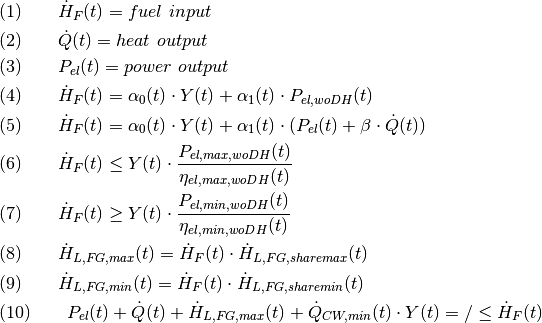where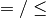depends on the CHP being back pressure or not.

The coefficients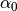and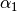can be determined given the efficiencies maximal/minimal load: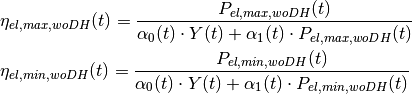Ifis given, e.g. for a motoric CHP: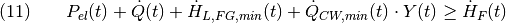The symbols used are defined as follows (with Variables (V) and Parameters (P)):

math. symbol attribute type explanation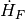H_F[n,t] V
input of enthalpy
through fuel inputP[n,t] V
provided
electric power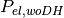P_woDH[n,t] V
electric power without
district heating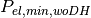P_min_woDH[n,t] P
min. electric power
without district heating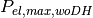P_max_woDH[n,t] P
max. electric power
without district heating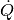Q[n,t] V provided heat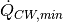Q_CW_min[n,t] P
minimal therm. condenserH_L_FG_min[n,t] V
flue gas enthalpy loss
at min heat extraction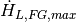H_L_FG_max[n,t] V
flue gas enthalpy loss
at max heat extraction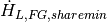H_L_FG_share_min[n,t] P
share of flue gas loss
at min heat extraction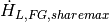H_L_FG_share_max[n,t] P
share of flue gas loss
at max heat extraction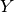Y[n,t] V
status variable
on/offn.alphas[n,t] P
coefficient
describing efficiencyn.alphas[n,t] P
coefficient
describing efficiencyBeta[n,t] P power loss index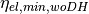Eta_el_min_woDH[n,t] P
el. eff. at min. fuel
flow w/o distr. heating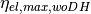Eta_el_max_woDH[n,t] P
el. eff. at max. fuel
flow w/o distr. heating

Note

See the GenericCHP class for all parameters and the mathematical background.

### GenericStorage (component)¶

A component to model a storage with its basic characteristics. The GenericStorage is designed for one input and one output. The nominal_storage_capacity of the storage signifies the storage capacity. You can either set it to the net capacity or to the gross capacity and limit it using the min/max attribute. To limit the input and output flows, you can define the nominal_value in the Flow objects. Furthermore, an efficiency for loading, unloading and a loss rate can be defined.

solph.GenericStorage(
label='storage',
inputs={b_el: solph.Flow(nominal_value=9, variable_costs=10)},
outputs={b_el: solph.Flow(nominal_value=25, variable_costs=10)},
loss_rate=0.001, nominal_storage_capacity=50,
inflow_conversion_factor=0.98, outflow_conversion_factor=0.8)


For initialising the state of charge before the first time step (time step zero) the parameter initial_storage_level (default value: None) can be set by a numeric value as fraction of the storage capacity. Additionally the parameter balanced (default value: True) sets the relation of the state of charge of time step zero and the last time step. If balanced=True, the state of charge in the last time step is equal to initial value in time step zero. Use balanced=False with caution as energy might be added to or taken from the energy system due to different states of charge in time step zero and the last time step. Generally, with these two parameters four configurations are possible, which might result in different solutions of the same optimization model:

• initial_storage_level=None, balanced=True (default setting): The state of charge in time step zero is a result of the optimization. The state of charge of the last time step is equal to time step zero. Thus, the storage is not violating the energy conservation by adding or taking energy from the system due to different states of charge at the beginning and at the end of the optimization period.
• initial_storage_level=0.5, balanced=True: The state of charge in time step zero is fixed to 0.5 (50 % charged). The state of charge in the last time step is also constrained by 0.5 due to the coupling parameter balanced set to True.
• initial_storage_level=None, balanced=False: Both, the state of charge in time step zero and the last time step are a result of the optimization and not coupled.
• initial_storage_level=0.5, balanced=False: The state of charge in time step zero is constrained by a given value. The state of charge of the last time step is a result of the optimization.

The following code block shows an example of the storage parametrization for the second configuration:

solph.GenericStorage(
label='storage',
inputs={b_el: solph.Flow(nominal_value=9, variable_costs=10)},
outputs={b_el: solph.Flow(nominal_value=25, variable_costs=10)},
loss_rate=0.001, nominal_storage_capacity=50,
initial_storage_level=0.5, balanced=True,
inflow_conversion_factor=0.98, outflow_conversion_factor=0.8)


If you want to view the temporal course of the state of charge of your storage after the optimisation, you need to check the storage_content in the results:

from oemof.solph import processing, views
results = processing.results(om)
column_name = (('your_storage_label', 'None'), 'storage_content')
SC = views.node(results, 'your_storage_label')['sequences'][column_name]


The storage_content is the absolute value of the current stored energy. By calling:

views.node(results, 'your_storage_label')['scalars']


you get the results of the scalar values of your storage, e.g. the initial storage content before time step zero (init_content).

For more information see the definition of the GenericStorage class or check the example repository of oemof.

#### Using an investment object with the GenericStorage component¶

Based on the GenericStorage object the GenericInvestmentStorageBlock adds two main investment possibilities.

• Invest into the flow parameters e.g. a turbine or a pump
• Invest into capacity of the storage e.g. a basin or a battery cell

Investment in this context refers to the value of the variable for the ‘nominal_value’ (installed capacity) in the investment mode.

As an addition to other flow-investments, the storage class implements the possibility to couple or decouple the flows with the capacity of the storage. Three parameters are responsible for connecting the flows and the capacity of the storage:

• invest_relation_input_capacity ‘ fixes the input flow investment to the capacity investment. A ratio of ‘1’ means that the storage can be filled within one time-period.
• invest_relation_output_capacity ‘ fixes the output flow investment to the capacity investment. A ratio of ‘1’ means that the storage can be emptied within one period.
• invest_relation_input_output ‘ fixes the input flow investment to the output flow investment. For values <1, the input will be smaller and for values >1 the input flow will be larger.

You should not set all 3 parameters at the same time, since it will lead to overdetermination.

The following example pictures a Pumped Hydroelectric Energy Storage (PHES). Both flows and the storage itself (representing: pump, turbine, basin) are free in their investment. You can set the parameters to None or delete them as None is the default value.

solph.GenericStorage(
label='PHES',
inputs={b_el: solph.Flow(investment= solph.Investment(ep_costs=500))},
outputs={b_el: solph.Flow(investment= solph.Investment(ep_costs=500)},
loss_rate=0.001,
inflow_conversion_factor=0.98, outflow_conversion_factor=0.8),
investment = solph.Investment(ep_costs=40))


The following example describes a battery with flows coupled to the capacity of the storage.

solph.GenericStorage(
label='battery',
inputs={b_el: solph.Flow()},
outputs={b_el: solph.Flow()},
loss_rate=0.001,
inflow_conversion_factor=0.98,
outflow_conversion_factor=0.8,
invest_relation_input_capacity = 1/6,
invest_relation_output_capacity = 1/6,
investment = solph.Investment(ep_costs=400))


Note

See the GenericStorage class for all parameters and the mathematical background.

### OffsetTransformer (component)¶

The OffsetTransformer object makes it possible to create a Transformer with different efficiencies in part load condition. For this object it is necessary to define the inflow as a nonconvex flow and to set a minimum load. The following example illustrates how to define an OffsetTransformer for given information for the output:

eta_min = 0.5       # efficiency at minimal operation point
eta_max = 0.8       # efficiency at nominal operation point
P_out_min = 20      # absolute minimal output power
P_out_max = 100     # absolute nominal output power

# calculate limits of input power flow
P_in_min = P_out_min / eta_min
P_in_max = P_out_max / eta_max

# calculate coefficients of input-output line equation
c1 = (P_out_max-P_out_min)/(P_in_max-P_in_min)
c0 = P_out_max - c1*P_in_max

# define OffsetTransformer
solph.custom.OffsetTransformer(
label='boiler',
inputs={bfuel: solph.Flow(
nominal_value=P_in_max,
max=1,
min=P_in_min/P_in_max,
nonconvex=solph.NonConvex())},
outputs={bth: solph.Flow()},
coefficients = [c0, c1])


This example represents a boiler, which is supplied by fuel and generates heat. It is assumed that the nominal thermal power of the boiler (output power) is 100 (kW) and the efficiency at nominal power is 80 %. The boiler cannot operate under 20 % of nominal power, in this case 20 (kW) and the efficiency at that part load is 50 %. Note that the nonconvex flow has to be defined for the input flow. By using the OffsetTransformer a linear relation of in- and output power with a power dependent efficiency is generated. The following figures illustrate the relations:Now, it becomes clear, why this object has been named OffsetTransformer. The linear equation of in- and outflow does not hit the origin, but is offset. By multiplying the Offset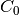with the binary status variable of the nonconvex flow, the origin (0, 0) becomes part of the solution space and the boiler is allowed to switch off: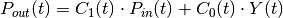Variables (V) and Parameters (P)
symbol attribute type explanation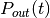flow[n, o, t] V Power of output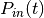flow[i, n, t] V Power of input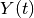status[i, n, t] V binary status variable of nonconvex input flow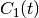coefficients[n, t] P linear coefficient 1 (slope)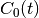coefficients[n, t] P linear coefficient 0 (y-intersection)

The following figures shows the efficiency dependent on the output power, which results in a nonlinear relation: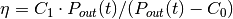The parametersand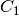can be given by scalars or by series in order to define a different efficiency equation for every timestep.

Note

See the OffsetTransformer class for all parameters and the mathematical background.

### ElectricalLine (custom)¶

Electrical line.

Note

See the ElectricalLine class for all parameters and the mathematical background.

### GenericCAES (custom)¶

Compressed Air Energy Storage (CAES). The following constraints describe the CAES: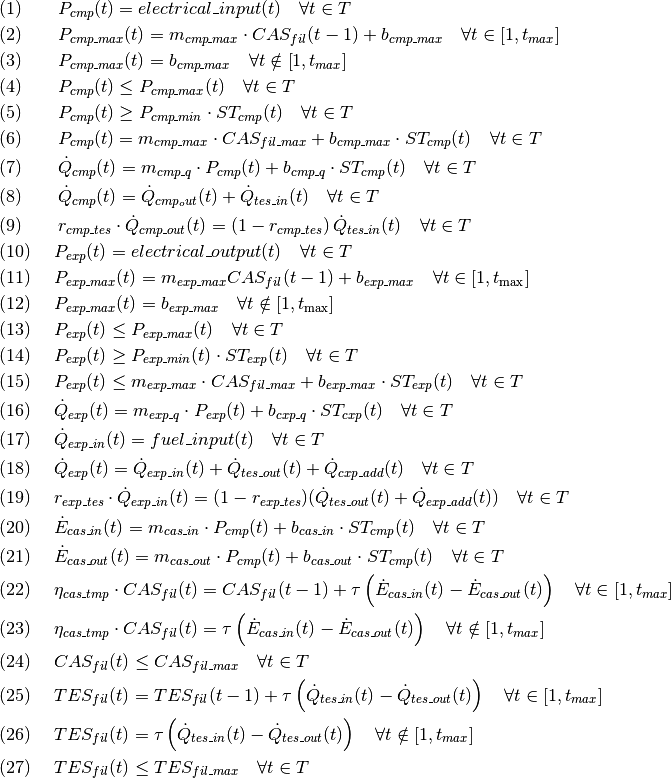Table: Symbols and attribute names of variables and parameters

Variables (V) and Parameters (P)
symbol attribute type explanation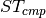cmp_st[n,t] V Status of compression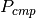cmp_p[n,t] V Compression power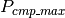cmp_p_max[n,t] V Max. compression power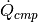cmp_q_out_sum[n,t] V
Summed
heat flow in compression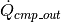cmp_q_waste[n,t] V Waste heat flow from compression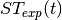exp_st[n,t] V Status of expansion (binary)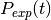exp_p[n,t] V Expansion power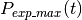exp_p_max[n,t] V Max. expansion power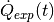exp_q_in_sum[n,t] V Summed heat flow in expansion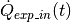exp_q_fuel_in[n,t] V Heat (external) flow into expansion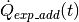exp_q_add_in[n,t] V Additional heat flow into expansion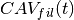cav_level[n,t] V
Filling level
if CAE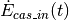cav_e_in[n,t] V Exergy flow into CAS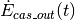cav_e_out[n,t] V Exergy flow from CAS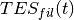tes_level[n,t] V Filling level of Thermal Energy Storage (TES)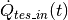tes_e_in[n,t] V
Heat
flow into TES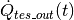tes_e_out[n,t] V
Heat
flow from TES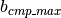cmp_p_max_b[n,t] P
Specific
y-intersection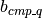cmp_q_out_b[n,t] P Specific y-intersection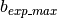exp_p_max_b[n,t] P Specific y-intersectionexp_q_in_b[n,t] P Specific y-intersection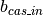cav_e_in_b[n,t] P Specific y-intersection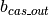cav_e_out_b[n,t] P Specific y-intersection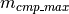cmp_p_max_m[n,t] P
Specific
slope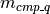cmp_q_out_m[n,t] P
Specific
slope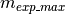exp_p_max_m[n,t] P
Specific
slope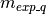exp_q_in_m[n,t] P
Specific
slope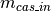cav_e_in_m[n,t] P
Specific
slope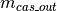cav_e_out_m[n,t] P
Specific
slope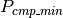cmp_p_min[n,t] P Min. compression power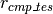cmp_q_tes_share[n,t] P
Ratio
between waste heat flow and heat flow into TES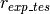exp_q_tes_share[n,t] P
Ratio
between external heat flow into expansion and heat flows from TES and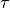m.timeincrement[n,t] P
Time interval
length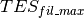tes_level_max[n,t] P Max. filling level of TES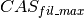cav_level_max[n,t] P
Max.
filling level of TEScav_eta_tmp[n,t] P
Temporal efficiency
(loss factor to take intertemporal losses into account)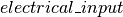flow[list(n.electrical_input.keys()), n, t] P Electr. power input into compression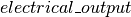flow[n, list(n.electrical_output.keys()), t] P Electr. power output of expansion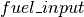flow[list(n.fuel_input.keys()), n, t] P
Heat input
(external) into Expansion

Note

See the GenericCAES class for all parameters and the mathematical background.

### SinkDSM (custom)¶

SinkDSM can used to represent flexibility in a demand time series. It can represent both, load shifting or load shedding. For load shifting, elasticity of the demand is described by upper (~oemof.solph.custom.sink_dsm.SinkDSM.capacity_up) and lower (~oemof.solph.custom.SinkDSM.capacity_down) bounds where within the demand is allowed to vary. Upwards shifted demand is then balanced with downwards shifted demand. For load shedding, shedding capability is described by ~oemof.solph.custom.SinkDSM.capacity_down. It both, load shifting and load shedding are allowed, ~oemof.solph.custom.SinkDSM.capacity_down limits the sum of both downshift categories.

SinkDSM provides three approaches how the Demand-Side Management (DSM) flexibility is represented in constraints It can be used for both, dispatch and investments modeling.

Cost can be associated to either demand up shifts or demand down shifts or both.

This small example of PV, grid and SinkDSM shows how to use the component

# Create some data
pv_day = [(-(1 / 6 * x ** 2) + 6) / 6 for x in range(-6, 7)]
pv_ts =  * 6 + pv_day +  * 6
data_dict = {"demand_el":  * len(pv_ts),
"pv": pv_ts,
"Cap_up": [0.5] * len(pv_ts),
"Cap_do": [0.5] * len(pv_ts)}
data = pd.DataFrame.from_dict(data_dict)

# Do timestamp stuff
datetimeindex = pd.date_range(start='1/1/2013', periods=len(data.index), freq='H')
data['timestamp'] = datetimeindex
data.set_index('timestamp', inplace=True)

# Create Energy System
es = solph.EnergySystem(timeindex=datetimeindex)
Node.registry = es

# Create bus representing electricity grid
b_elec = solph.Bus(label='Electricity bus')

# Create a back supply
grid = solph.Source(label='Grid',
outputs={
b_elec: solph.Flow(
nominal_value=10000,
variable_costs=50)}
)

# PV supply from time series
s_wind = solph.Source(label='wind',
outputs={
b_elec: solph.Flow(
fix=data['pv'],
nominal_value=3.5)}
)

# Create DSM Sink
demand_dsm = solph.custom.SinkDSM(label="DSM",
inputs={b_elec: solph.Flow()},
demand=data['demand_el'],
capacity_up=data["Cap_up"],
capacity_down=data["Cap_do"],
delay_time=6,
max_demand=1,
max_capacity_up=1,
max_capacity_down=1,
approach="DIW",
cost_dsm_down=5)


Yielding the following resultsNote

• Keyword argument method from v0.4.1 has been renamed to approach in v0.4.2 and methods have been renamed.
• The parameters demand, capacity_up and capacity_down have been normalized to allow investments modeling. To retreive the original dispatch behaviour from v0.4.1, set max_demand=1, max_capacity_up=1, max_capacity_down=1.
• This component is a candidate component. It’s implemented as a custom component for users that like to use and test the component at early stage. Please report issues to improve the component.
• See the SinkDSM class for all parameters and the mathematical background.

## Using the investment mode¶

As described in Optimise your energy system the typical way to optimise an energy system is the dispatch optimisation based on marginal costs. Solph also provides a combined dispatch and investment optimisation. Based on investment costs you can compare the usage of existing components against building up new capacity. The annual savings by building up new capacity must therefore compensate the annuity of the investment costs (the time period does not have to be one year but depends on your Datetime index).

See the API of the Investment class to see all possible parameters.

Basically an instance of the investment class can be added to a Flow or a Storage. All parameters that usually refer to the nominal_value/capacity will now refer to the investment variables and existing capacity. It is also possible to set a maximum limit for the capacity that can be build. If existing capacity is considered for a component with investment mode enabled, the ep_costs still apply only to the newly built capacity.

The investment object can be used in Flows and some components. See the Solph components section for detailed information of each component.

For example if you want to find out what would be the optimal capacity of a wind power plant to decrease the costs of an existing energy system, you can define this model and add an investment source. The wind_power_time_series has to be a normalised feed-in time series of you wind power plant. The maximum value might be caused by limited space for wind turbines.

solph.Source(label='new_wind_pp', outputs={electricity: solph.Flow(
fix=wind_power_time_series,
investment=solph.Investment(ep_costs=epc, maximum=50000))})


Let’s slightly alter the case and consider for already existing wind power capacity of 20,000 kW. We’re still expecting the total wind power capacity, thus we allow for 30,000 kW of new installations and formulate as follows.

solph.Source(label='new_wind_pp', outputs={electricity: solph.Flow(
fix=wind_power_time_series,
investment=solph.Investment(ep_costs=epc,
maximum=30000,
existing=20000))})


The periodical costs (ep_costs) are typically calculated as follows:

capex = 1000  # investment cost
lifetime = 20  # life expectancy
wacc = 0.05  # weighted average of capital cost
epc = capex * (wacc * (1 + wacc) ** lifetime) / ((1 + wacc) ** lifetime - 1)


This also implemented in the annuity function of the economics module in the oemof.tools package. The code above would look like this:

from oemof.tools import economics
epc = economics.annuity(1000, 20, 0.05)


So far, the investment costs and the installed capacity are mathematically a line through origin. But what if there is a minimum threshold for doing an investment, e.g. you cannot buy gas turbines lower than a certain nominal power, or, the marginal costs of bigger plants decrease. Therefore, you can use the parameter nonconvex and offset of the investment class. Both, work with investment in flows and storages. Here is an example of an transformer:

trafo = solph.Transformer(
label='transformer_nonconvex',
inputs={bus_0: solph.Flow()},
outputs={bus_1: solph.Flow(
investment=solph.Investment(
ep_costs=4,
maximum=100,
minimum=20,
nonconvex=True,
offset=400))},
conversion_factors={bus_1: 0.9})


In this examples, it is assumed, that independent of the size of the transformer, there are always fix investment costs of 400 (€). The minimum investment size is 20 (kW) and the costs per installed unit are 4 (€/kW). With this option, you could theoretically approximate every cost function you want. But be aware that for every nonconvex investment flow or storage you are using, an additional binary variable is created. This might boost your computing time into the limitless.

The following figures illustrates the use of the nonconvex investment flow. Here,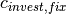is the offset value and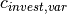is the ep_costs value:In case of a convex investment (which is the default setting nonconvex=Flase), the minimum attribute leads to a forced investment, whereas in the nonconvex case, the investment can become zero as well.

The calculation of the specific costs per kilowatt installed capacity results in the following relation for convex and nonconvex investments:See InvestmentFlow and GenericInvestmentStorageBlock for all the mathematical background, like variables and constraints, which are used.

Note

At the moment the investment class is not compatible with the MIP classes NonConvex.

## Mixed Integer (Linear) Problems¶

Solph also allows you to model components with respect to more technical details such as a minimal power production. Therefore, the class NonConvex exists in the options module. Note that the usage of this class is currently not compatible with the Investment class.

If you want to use the functionality of the options-module, the only thing you have to do is to invoke a class instance inside your Flow() - declaration:

b_gas = solph.Bus(label='natural_gas')
b_el = solph.Bus(label='electricity')
b_th = solph.Bus(label='heat')

solph.Transformer(
label='pp_chp',
inputs={b_gas: Flow()},
outputs={b_el: Flow(nominal_value=30,
min=0.5,
nonconvex=NonConvex()),
b_th: Flow(nominal_value=40)},
conversion_factors={b_el: 0.3, b_th: 0.4})


The NonConvex() object of the electrical output of the created LinearTransformer will create a ‘status’ variable for the flow. This will be used to model for example minimal/maximal power production constraints if the attributes min/max of the flow are set. It will also be used to include start up constraints and costs if corresponding attributes of the class are provided. For more information see the API of the NonConvex class and its corresponding block class NonConvexFlow.

Note

The usage of this class can sometimes be tricky as there are many interdenpendencies. So check out the examples and do not hesitate to ask the developers if your model does not work as expected.

You can add additional constraints to your Model. See flexible_modelling in the example repository to learn how to do it.

Some predefined additional constraints can be found in the constraints module.

## The Grouping module (Sets)¶

To construct constraints, variables and objective expressions inside all Block classes and the models modules, so called groups are used. Consequently, certain constraints are created for all elements of a specific group. Thus, mathematically the groups depict sets of elements inside the model.

The grouping is handled by the solph grouping module groupings which is based on the groupings module functionality of oemof network. You do not need to understand how the underlying functionality works. Instead, checkout how the solph grouping module is used to create groups.

The simplest form is a function that looks at every node of the energy system and returns a key for the group depending e.g. on node attributes:

 def constraint_grouping(node):
if isinstance(node, Bus) and node.balanced:
return blocks.Bus
if isinstance(node, Transformer):
return blocks.Transformer
GROUPINGS = [constraint_grouping]


This function can be passed in a list to groupings of oemof.solph.network.energy_system.EnergySystem. So that we end up with two groups, one with all Transformers and one with all Buses that are balanced. These groups are simply stored in a dictionary. There are some advanced functionalities to group two connected nodes with their connecting flow and others (see for example: FlowsWithNodes class in the oemof.network package).

## Using the Excel (csv) reader¶

Alternatively to a manual creation of energy system component objects as describe above, can also be created from a excel sheet (libreoffice, gnumeric…).

The idea is to create different sheets within one spreadsheet file for different components. Afterwards you can loop over the rows with the attributes in the columns. The name of the columns may differ from the name of the attribute. You may even create two sheets for the GenericStorage class with attributes such as C-rate for batteries or capacity of turbine for a PHES.

Once you have create your specific excel reader you can lower the entry barrier for other users. It is some sort of a GUI in form of platform independent spreadsheet software and to make data and models exchangeable in one archive.

See oemof’s example repository for an excel reader example.

## Handling Results¶

The main purpose of the processing module is to collect and organise results. The views module will provide some typical representations of the results. Plots are not part of solph, because plots are highly individual. However, the provided pandas.DataFrames are a good start for plots. Some basic functions for plotting of optimisation results can be found in the separate repository oemof_visio.

The processing.results function gives back the results as a python dictionary holding pandas Series for scalar values and pandas DataFrames for all nodes and flows between them. This way we can make use of the full power of the pandas package available to process the results.

See the pandas documentation to learn how to visualise, read or write or how to access parts of the DataFrame to process them.

The results chapter consists of three parts:

The first step is the processing of the results (Collecting results) This is followed by basic examples of the general analysis of the results (General approach) and finally the use of functionality already included in solph for providing a quick access to your results (Easy access). Especially for larger energy systems the general approach will help you to write your own results processing functions.

### Collecting results¶

Collecting results can be done with the help of the processing module. A solved model is needed:

[...]
model.solve(solver=solver)
results = solph.processing.results(model)


The scalars and sequences describe nodes (with keys like (node, None)) and flows between nodes (with keys like (node_1, node_2)). You can directly extract the data in the dictionary by using these keys, where “node” is the name of the object you want to address. Processing the results is the prerequisite for the examples in the following sections.

### General approach¶

As stated above, after processing you will get a dictionary with all result data. If you want to access your results directly via labels, you can continue with Easy access. For a systematic analysis list comprehensions are the easiest way of filtering and analysing your results.

The keys of the results dictionary are tuples containing two nodes. Since flows have a starting node and an ending node, you get a list of all flows by filtering the results using the following expression:

flows = [x for x in results.keys() if x is not None]


On the same way you can get a list of all nodes by applying:

nodes = [x for x in results.keys() if x is None]


Probably you will just get storages as nodes, if you have some in your energy system. Note, that just nodes containing decision variables are listed, e.g. a Source or a Transformer object does not have decision variables. These are in the flows from or to the nodes.

All items within the results dictionary are dictionaries and have two items with ‘scalars’ and ‘sequences’ as keys:

for flow in flows:
print(flow)
print(results[flow]['scalars'])
print(results[flow]['sequences'])


There many options of filtering the flows and nodes as you prefer. The following will give you all flows which are outputs of transformer:

flows_from_transformer = [x for x in flows if isinstance(
x, solph.Transformer)]


You can filter your flows, if the label of in- or output contains a given string, e.g.:

flows_to_elec = [x for x in results.keys() if 'elec' in x.label]


Getting all labels of the starting node of your investment flows:

flows_invest = [x.label for x in flows if hasattr(
results[x]['scalars'], 'invest')]


### Easy access¶

The solph package provides some functions which will help you to access your results directly via labels, which is helpful especially for small energy systems. So, if you want to address objects by their label, you can convert the results dictionary such that the keys are changed to strings given by the labels:

views.convert_keys_to_strings(results)
print(results[('wind', 'bus_electricity')]['sequences']


Another option is to access data belonging to a grouping by the name of the grouping (note also this section on groupings. Given the label of an object, e.g. ‘wind’ you can access the grouping by its label and use this to extract data from the results dictionary.

node_wind = energysystem.groups['wind']
print(results[(node_wind, bus_electricity)])


However, in many situations it might be convenient to use the views module to collect information on a specific node. You can request all data related to a specific node by using either the node’s variable name or its label:

data_wind = solph.views.node(results, 'wind')


A function for collecting and printing meta results, i.e. information on the objective function, the problem and the solver, is provided as well:

meta_results = solph.processing.meta_results(om)
pp.pprint(meta_results)